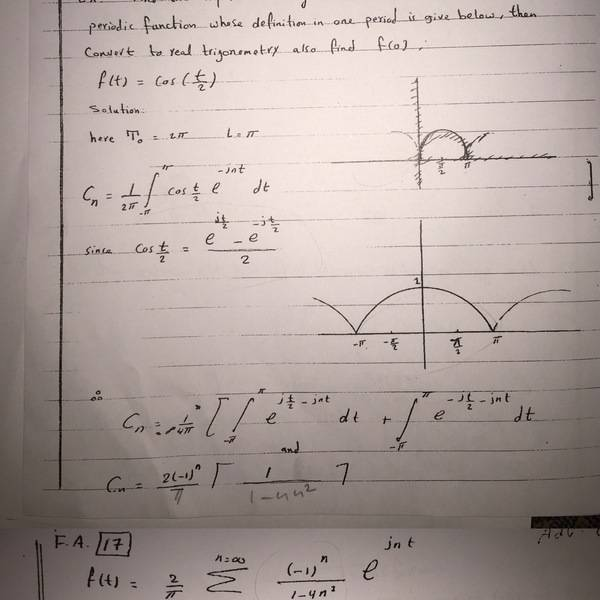# Complex Fourier Series HW

Aows

## Homework Statement

Q:/ Find the complex form of fourier series for the following periodic function whose definition in one period is given below then convert to real trigonometry also find f(0).
f(t)=cos(t/2), notes: (T=2*pi) (L=pi)

## Homework Equations

1) f(t)=sum from -inf to +inf (Cn exp(j*n*(pi/L)*t)
2) Cn=(1/2pi) *integration from -L to +L (f(t) exp (-j * n (pi/L)* t) *dt

## The Attempt at a Solution

i failed at finding the solution to the Cn coefficient

stevendaryl
Staff Emeritus

## Homework Statement

Q:/ Find the complex form of fourier series for the following periodic function whose definition in one period is given below then convert to real trigonometry also find f(0).
f(t)=cos(t/2), notes: (T=2*pi) (L=pi)

## Homework Equations

1) f(t)=sum from -inf to +inf (Cn exp(j*n*(pi/L)*t)
2) Cn=(1/2pi) *integration from -L to +L (f(t) exp (-j * n (pi/L)* t) *dt

## The Attempt at a Solution

i failed at finding the solution to the Cn coefficient

Well, post what the equations look like when you substitute $cos(t/2)$ in for $f(t)$. Also, for evaluating the integral, it might help to convert it to exponentials, using:

$cos(x) = \frac{1}{2} (e^{i x} + e^{-ix})$

Aows
Well, post what the equations look like when you substitute $cos(t/2)$ in for $f(t)$. Also, for evaluating the integral, it might help to convert it to exponentials, using:

$cos(x) = \frac{1}{2} (e^{i x} + e^{-ix})$
Hello,
what do you want me to post ?

Aows
here is part of the question